# Solving Mathematical Representations of Free-Body Diagrams

Instructor: Matthew Bergstresser
A free-body diagram is a visual way to represent the forces acting on a mass. This lesson will go through how to set up and solve the mathematical expressions required to determine the acceleration of the mass.

## From Free-Body Diagram to Solution

Drawing a free-body diagram is the first step in determining the acceleration of a mass using Newton's second law: Σ F = ma. Sometimes, a problem will provide the free-body diagram for you, and your job is to write the mathematical expressions including the forces from the diagram to solve for the acceleration.

The first thing to consider before writing mathematical expressions from a free-body diagram is how many dimensions are involved? Each axis must have its own mathematical expression because force is a vector. Vectors have a specific direction associated with them, and only like directions can be summed.

Let's start with an example where the free-body diagram (FBD) is provided and we have to turn the information in the diagram into a mathematical expression.

### Example 1

Prompt: Use the FBD to determine two mathematical expressions for a, one in the x-direction, and one in the y-direction. Then describe how to use the x and y accelerations to get the overall (net) acceleration.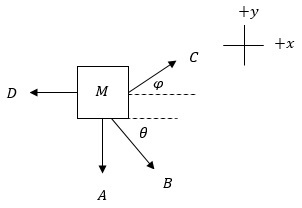Solution: In our example there are only 2 axes therefore 2 dimensions.

Any forces that are not parallel to a pure direction (parallel to the x-axis, y-axis, or z-axis) must be multiplied by a trigonometric function of its related angle. This will get the magnitude of the force in the pure directions, x and y. Now we are ready to turn FBD 1 into a mathematical expression.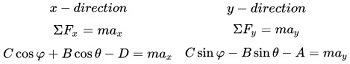Now we can determine the x and y accelerations. Let's use algebra to get each expression solved for the accelerations.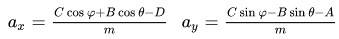To get the net acceleration we have to set up a right triangle and use the Pythagorean theorem. Since this example doesn't include numbers, let's say that its x-acceleration is positive, and its y-acceleration is negative.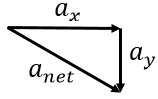Now, let's do an example where we use numbers to determine an acceleration.

### Example 2

Prompt: Use the FBD to determine the acceleration of the 5 kg mass.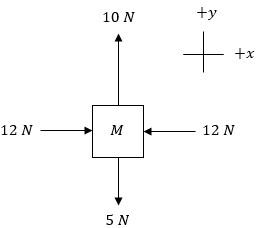Solution: First we break the vectors up into the respective directions, and then solve for the acceleration in each direction, using 5 kg for m.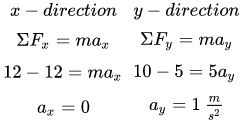The mass accelerates in the positive y-direction at 1 m/s2 .

Physics requires a lot of practice, so we will do another example.

### Example 3

Prompt: The FBD shown is for a 10 kg mass sliding up a ramp ( θ = 30o ) and friction is acting on the mass. The friction force is equal to μ = 0.4 times the normal force, and the normal force is mgcosθ . Determine the acceleration of the mass.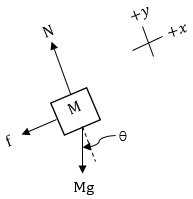• f is the friction force
• N is the normal force
• M is the mass
• g is the acceleration due to gravity ( 9.8 m/s2 )

To unlock this lesson you must be a Study.com Member.

### Register to view this lesson

Are you a student or a teacher?

### Unlock Your Education

#### See for yourself why 30 million people use Study.com

##### Become a Study.com member and start learning now.
Back
What teachers are saying about Study.com

### Earning College Credit

Did you know… We have over 160 college courses that prepare you to earn credit by exam that is accepted by over 1,500 colleges and universities. You can test out of the first two years of college and save thousands off your degree. Anyone can earn credit-by-exam regardless of age or education level.